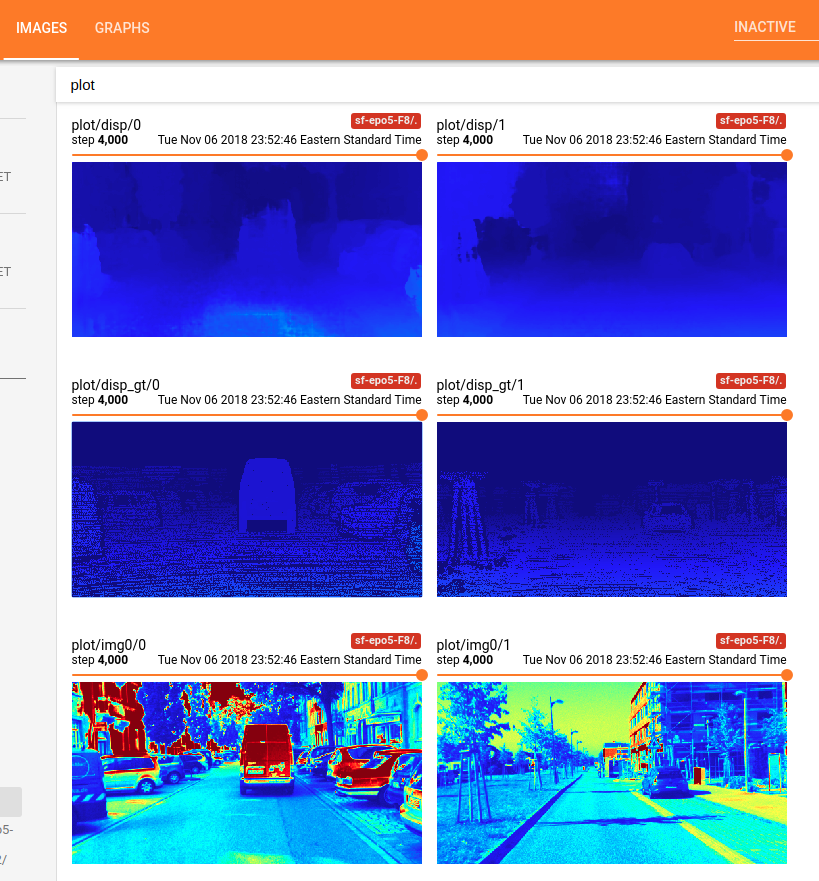# How to display custom images in TensorBoard using Keras?

07 Nov 2018

## How to display custom images in TensorBoard using Keras?

Check my answer (https://stackoverflow.com/a/53183922/3613060) to this question at Stackoverflow.

I provide the following code to finish the following things using TensorBoard in Keras:

• problem setup: to predict the disparity map in binocular stereo matching;
• to feeds the model with input left image x and ground truth disparity map gt;
• to display the input x and ground truth ‘gt’, at some iteration time;
• to display the output y of your model, at some iteration time.

1) First of all, you have to make your costumed callback class with Callback. Note that a callback has access to its associated model through the class property self.model. Also Note: you have to feed the input to the model with feed_dict, if you want to get and display the output of your model.


from keras.callbacks import Callback
import numpy as np
from keras import backend as K
import tensorflow as tf

# make the 1 channel input image or disparity map look good within this color map. This function is not necessary for this Tensorboard problem shown as above. Just a function used in my own research project.
def colormap_jet(img):
return cv2.cvtColor(cv2.applyColorMap(np.uint8(img), 2), cv2.COLOR_BGR2RGB)

class customModelCheckpoint(Callback):
def __init__(self, log_dir = './logs/tmp/', feed_inputd_display = None):
super(customModelCheckpoint, self).__init__()
self.seen = 0
self.feed_inputs_display = feed_inputs_display
self.writer = tf.summary.FileWriter(log_dir)

# this function will return the feeding data for TensorBoard visualization;
# arguments:
#  * feed_input_display : [(input_yourModelNeed, left_image, disparity_gt ), ..., (input_yourModelNeed, left_image, disparity_gt), ...], i.e., the list of tuples of Numpy Arrays what your model needs as input and what you want to display using TensorBoard. Note: you have to feed the input to the model with feed_dict, if you want to get and display the output of your model.
def custom_set_feed_input_to_display(self, feed_inputs_display):
self.feed_inputs_display = feed_inputs_display

# copied from the above answers;
def make_image(self, numpy_img):
from PIL import Image
height, width, channel = numpy_img.shape
image = Image.fromarray(numpy_img)
import io
output = io.BytesIO()
image.save(output, format='PNG')
image_string = output.getvalue()
output.close()
return tf.Summary.Image(height=height, width=width, colorspace= channel, encoded_image_string=image_string)

# A callback has access to its associated model through the class property self.model.
def on_batch_end(self, batch, logs = None):
logs = logs or {}
self.seen += 1
if self.seen % 200 == 0: # every 200 iterations or batches, plot the costumed images using TensorBorad;
summary_str = []
for i in range(len(self.feed_inputs_display)):
feature, disp_gt, imgl = self.feed_inputs_display[i]
disp_pred = np.squeeze(K.get_session().run(self.model.output, feed_dict = {self.model.input : feature}), axis = 0)
#disp_pred = np.squeeze(self.model.predict_on_batch(feature), axis = 0)
summary_str.append(tf.Summary.Value(tag= 'plot/img0/{}'.format(i), image= self.make_image( colormap_jet(imgl)))) # function colormap_jet(), defined above;
summary_str.append(tf.Summary.Value(tag= 'plot/disp_gt/{}'.format(i), image= self.make_image( colormap_jet(disp_gt))))
summary_str.append(tf.Summary.Value(tag= 'plot/disp/{}'.format(i), image= self.make_image( colormap_jet(disp_pred))))



2.) Next, pass this callback object to fit_generator() for your model, like:

   feed_inputs_4_display = some_function_you_wrote()
callback_mc = customModelCheckpoint( log_dir = log_save_path, feed_inputd_display = feed_inputs_4_display)
# or
callback_mc.custom_set_feed_input_to_display(feed_inputs_4_display)
yourModel.fit_generator(... callbacks = callback_mc)
...


3.) Now your can run the code, and go the TensorBoard host to see the costumed image display. For example, this is what I got using the aforementioned code:Done! Enjoy!